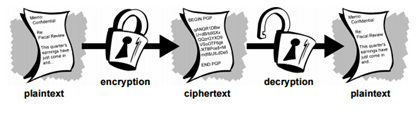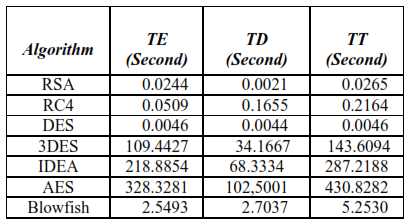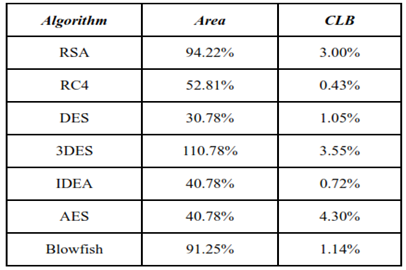Monday, March 23, 2015

Hardware Implementation of Cryptography techniques

When Julius Caesar sent information to his generals, he never believed his messengers. So he interchanges every A with a D, every B with an E, and so on through the alphabet in his message. Only authenticated person who knew the key could decipher his messages. By doing this only authenticated person could understand his message. This is somewhat called cryptography.

Cryptography: - Cryptography is a science and study of secret writing. It is a method in which data is stored and transmitted in a specific form so that only those for whom it is proposed can read and practice it. Following figure shows it in simple way.Fig- Cryptography process

Plaintext- Original Data to be transmitted.
Ciphertext - Modified or encrypted data.

Cryptography key: - By using key, one can decrypt the ciphertext and get original data. Two types of key are there, public key and private key.

An overview of some cryptographic techniques is given below:-

1. RSA algorithm

RSA uses a public key and a private key. The public key is designed for everyone to encrypt a original data. The original data which is to be transmitted is encrypted using public key and by using private key it can be decoded in a reasonable amount of time. If a message M is transmitted than ciphertext would be
C=ME mod n.

Where E is public key and n is product of two prime numbers.

2. RC4 algorithm

It is a stream cipher with byte oriented operations whose key size can be varied. Random permutations are used in this algorithm for encryption and decryption process. Length of the key can be in between 1 and 256 bytes to initialize a 256-byte state table. This state table is used to generate a sequence of pseudo random bytes and a stream. By xoring this stream with the plaintext, we get a ciphertext. Each and every element in the state table must be swapped at least once. Limitation of RC4 is that in every 256 keys there is one weak key.

3. DES algorithm

Data Encryption Standard (DES) is a symmetric key algorithm that encrypt 64-bit blocks of plaintext into 64-bit of ciphertext by using 56-bit long keys. The encryption process completes in 16 rounds. DES encryption/decryption times are faster than those of RC4 encryption/decryption times.  DES is used to secure confidential information of smart cards and ciphering of images and so on.

4. 3DES algorithm

It is also Symmetric Key Algorithms. It is called Triple  DES(3DES) because it encrypts  original data  three times as compared to DES, first encrypted it with 56-bit key K by applying DES encryption and then applying DES decryption  with 56-bit key K2 final applying DES 56-bit key K1. Same operation takes place for Decryption but in reverse order.

5. IDEA algorithm

IDEA (international data encryption algorithm) operates on 64-bit blocks and uses a key of length 128-bit, and consists of a series of eight similar rounds or stages and a half output stage. Thus a total of 8 and half rounds are needed to encrypt message. IDEA is a much secure method of encryption because of its different operations such as- modular addition and multiplication, and bitwise exclusive OR (XOR) — which are algebraically incompatible in some manner. It used in steganography and other applications.

6. AES algorithm

AES is Symmetric Key Algorithms. Three different keys of different sizes can be used in this algorithm such as: 128, 192, or 256 bits long keys. If we use 128 bit key than 10 rounds are needed for encryption, if 192-bit key is used than 12 rounds needed, and for 256-bit keys, 14 rounds needed. AES is more protected than DES and 3DES, as this algorithm uses longer length key. AES provides faster encryption than DES and 3DES, and this feature of AES makes it ideal for software applications and those of hardware which require low-latency or high throughput.

7. Blowfish algorithm

Blowfish is a symmetric block cipher just like DES or IDEA. It takes a variable-length key. It’s key length could be in between 32 to 448 bits. It is designed such as it is a speedy alternative to that of existing cryptographic algorithms.

Comparison in terms of encryption time(TE), decryption time(TD) and total time(TT) as per literature surveyComparison of encryption algorithms on the bases of hardware implementation as per the literature survey